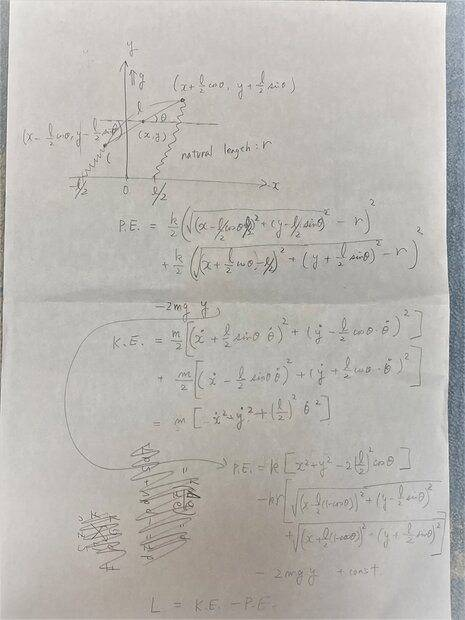# Interpretation of Lagrangian solution (complex numbers)

• A
• Mishal0488

#### Mishal0488

Hi Guys

Finally after a great struggle I have managed to develop and solve my Lagrangian system. I have checked it numerous times over and over and I believe that everything is correct.

I have attached a PDF which has the derivation of the system. Please ignore all the forcing functions and damping for the system.

Essentially I have taken the holonomic constraint and represented (r+b) which I have taken as "B" as the subject of the formula, there after I am substituting the result into the derived differential equations.

The Matlab code is presented below, the "Statespace6" function is the representation of the differential equations while the main code is used to execute the code. There a number of plots developed (Please don't laugh at some of my spelling mistakes, I am going to fix these up later). The results do not make sense to me for the theta2 and "b" component of the system. In some instances there are imaginary numbers which are developed, which I am not sure how to interpret everything. Filtering in the results in most cases presents almost realistic results, however I am not completely convinced that the system is correct.

Further to this, the results which are understandable/realistic are developed when the same initial positions are imposed for "a" and "b" with regards to the radial displacement.

It would be nice to get an opinion of what I have done, and gather some comments, input and advice so I know what to look into next with regards to fixing my solutions.

 function xp = Statespace6(t,x,r,L,k,m1,m2) theta1 = x(1); theta1_dot = x(2); theta2 = x(3); theta2_dot = x(4); a = x(5); a_dot = x(6); A = (a+r); %B R1 = 2*A*cos(theta1)*cos(theta2); R3 = 2*L*sin(theta2)+2*A*sin(theta1)*sin(theta2); one = -A^2+L^2; two = A^2*(cos(2*theta1)*cos(2*theta2)+sin(2*theta1)*sin(2*theta2)); three = -L^2*cos(2*theta2); four = -2*A*L*sin(theta1); five = -2*A*L*(sin(theta1)*cos(2*theta2)-cos(theta1)*sin(2*theta2)); R2 = sqrt(2)*sqrt(one+two+three+four+five); B = 0.5*(R1+R2+R3); %B = 0.5*(R1-R2+R3) %b_dot R1_dot = 2*(a_dot*cos(theta1)*cos(theta2) - A*theta1_dot*sin(theta1)*cos(theta2) - A*theta2_dot*cos(theta1)*sin(theta2)); R3_dot = 2*L*theta2_dot*cos(theta2) + 2*(a_dot*sin(theta1)*sin(theta2) + A*theta1_dot*cos(theta1)*sin(theta2) + A*sin(theta1)*theta2_dot*cos(theta2)); one_dot = -2*a_dot*A; two_dot = 2*a_dot*A*cos(2*theta1)*cos(2*theta2) + -A^2*2*theta1_dot*sin(2*theta1)*cos(2*theta2) + -A^2*cos(2*theta1)*2*theta2_dot*sin(2*theta2) + 2*a_dot*A*sin(2*theta1)*sin(2*theta2) + A^2*2*theta1_dot*cos(2*theta1)*sin(2*theta2) + A^2*sin(2*theta1)*2*theta2_dot*cos(2*theta2); three_dot = L^2*2*theta2_dot*sin(2*theta2); four_dot = -2*a_dot*L*sin(theta1)-2*A*L*theta1_dot*cos(theta1); five_dot = -2*a_dot*L*sin(theta1)*cos(2*theta2) - 2*A*L*theta1_dot*cos(theta1)*cos(2*theta2) - 2*A*L*sin(theta1)*2*theta1_dot*sin(2*theta2) + 2*a_dot*L*cos(theta1)*sin(2*theta2) - 2*A*L*theta1_dot*sin(theta1)*sin(2*theta2) + 2*A*L*cos(theta1)*2*theta2_dot*cos(2*theta2); R2_dot = sqrt(2)*(one_dot+two_dot+three_dot+four_dot+five_dot)/(2*sqrt(B)); b_dot = (R1_dot+R2_dot+R3_dot)/(4*sqrt(R1+R2+R3)); %differential equations %theta1 theta1_dotdot = (-2*m1*theta1_dot*a_dot*A-9.81*m1*sin(theta1)*A)/(m1*A^2); %theta2 theta2_dotdot = (-2*m2*theta2_dot*b_dot*B-9.81*m2*sin(theta2)*B)/(m2*B^2); %a a_dotdot = (m1*theta1_dot^2*A-k*a+9.81*m1*cos(theta1))/m1; %b b_dotdot = (m2*theta2_dot^2*B-k*(B-r)+9.81*m2*cos(theta2))/m2; xp = [x(2); theta1_dotdot; x(4); theta2_dotdot; x(6); a_dotdot; x(8);%b_dot; b_dotdot;]; end 

 clc; clear; r = 1; L = 2; k = 353160; m1 = 3500/2; m2 = 3500/2; inc = 0.0001; SolverOptions = odeset('RelTol',1e-5,'AbsTol',1e-5); [t,y] = ode45(@Statespace6,[0:inc:2],[0.1 0.1 0.1 0.1 0.1 0.1 0.1 0.1],SolverOptions,r,L,k,m1,m2); theta1 = y(:,1); theta1_dot = y(:,2); theta2 = y(:,3); theta2_dot = y(:,4); a = y(:,5); a_dot = y(:,6); %motion plots xx = (y(:,5)+r).*sin(y(:,1)); yy = (y(:,5)+r).*cos(y(:,1)); figure(1) hold on plot(xx,yy) title('Motion plot for mass1') hold off xx1 = (y(:,7)+r).*sin(y(:,3)); yy1 = (y(:,7)+r).*cos(y(:,3)); figure(2) hold on title('Motion plot for mass2 using unfiltered data') plot(xx1,yy1) hold off figure(4) hold on L1 = plot(t,y(:,8)) L2 = plot(t,a_dot) title('radial velocities using unfiltered data') legend([L1 L2],'b dot','a dot'); hold off figure(5) hold on L1 = plot(t,theta1) L2 = plot(t,theta2) title('Rotational displacement using unfiltered data') legend([L1 L2],'theta1','theta2'); hold off figure(6) hold on L1 = plot(t,y(:,5)) L2 = plot(t,y(:,7)) title('radial displacement using unfiltered data') legend([L1 L2],'a','b') hold off figure(7) hold on L1 = plot(t,y(:,2)) L2 = plot(t,y(:,4)) title('Angular velocity using unfiltered data') legend([L1 L2],'theta1 dot','theta2 dot') hold off %filter data Fs = 1/inc; %sampling rate Fc = 1/(Fs/2); %cut off frequency [b1 a1] = butter(4,Fc,'high'); b_new = filtfilt(b1,a1,y(:,7)); figure(8) hold on L1 = plot(t,b_new) L2 = plot(t,y(:,5)) legend([L1 L2],'b','a'); title('radial displacement filtereddata') hold off Fc = 1/(Fs/4); %cut off frequency [b1 a1] = butter(4,Fc,'high'); b_dotnew = filtfilt(b1,a1,y(:,8)); figure(9) hold on L1 = plot(t,b_dotnew) L2 = plot(t,y(:,6)) legend([L1 L2],'b dot','a dot'); title('radial velocity filtered data') hold off xx1 = (b_new+r).*sin(y(:,3)); yy1 = (b_new+r).*cos(y(:,3)); figure(10) hold on title('Motion plot for mass2 using filtered data') plot(xx1,yy1) hold off %holonomic constraint i = size(t); i = i(1,1); for j = 1:i H(j) = ((r+y(j,5))*sin(y(j,1))+L-(r+b_new(j))*sin(y(j,3)))^2+((r+y(j,5))*cos(y(j,1))-(r+b_new(j))*cos(y(j,3)))^2-L^2; end figure(11) hold on plot(t,H); title('Holonomic constraint using filtered data') hold off for j = 1:i H(j) = ((r+y(j,5))*sin(y(j,1))+L-(r+y(j,7))*sin(y(j,3)))^2+((r+y(j,5))*cos(y(j,1))-(r+y(j,7))*cos(y(j,3)))^2-L^2; end figure(12) hold on plot(t,H); title('Holonomic constraint using unfiltered data') hold off 

#### Attachments

• img20211008_13472537.pdf
2.5 MB · Views: 50•